# Introduction

This vignette assumes the reader is already familiar with using nimble to perform Markov chain Monte Carlo (MCMC).

The principal motivation of this package is that, when target posterior distributions are multimodal standard MCMC algorithms often perform badly. In these situations, standard algorithms often propose few, if any, jumps between modes, and can thus provide very poor approximations to target posterior distributions. The nimbleAPT package permits nimble users to perform adaptive parallel tempering (APT) as a potential solution for sampling multimodal posterior distributions.

Parallel tempering is an MCMC technique where the posterior likelihood (of a Bayesian model) is ‘heated’, ‘or tempered’ to various degrees. As temperature is increased, likelihoods become flatter and this can increase the probability and frequency of between-mode jumps. In practice, a “temperature ladder” (a ranked set of temperatures) is established, MCMC is performed within each rung of the temperature ladder, and a special between-rung MCMC step is introduced so that parameter sets can move up and down the temperature ladder. Bayesian inference is made on the posterior samples of the unheated rung only. Adaptive parallel tempering algorithms automatically tune the temperatures of the temperature ladder so that target acceptance rates for proposed between-rung jumps are achieved.

The nimbleAPT package provides the following…

1. A buildAPT function: adapted from nimble’s buildMCMC, this function sets up the APT algorithm, including between-rung steps and temperature ladder adaptation.
2. A set of samplers, adapted from nimble’s standard MCMC samplers, that include heating of the posterior likelihood.

# Toy example

The following toy problem illustrates how classic MCMC algorithms can struggle to approximate multimodal posteriors. The true posterior distribution for centroids should have four modes, but as we will see, classic samplers can struggle to explore such a posterior distribution.

First, we create a nimble model with a multimodal posterior. For this model there is no information in the data to remove uncertainty about the sign of the elements of centroids.

library(nimbleAPT) # Also loads nimble
#> nimble version 0.12.1 is loaded.
#> For more information on NIMBLE and a User Manual,
#> please visit https://R-nimble.org.
#>
#> Attaching package: 'nimble'
#> The following object is masked from 'package:stats':
#>
#>     simulate

bugsCode <- nimbleCode({
for (ii in 1:nObs) {
y[ii,1:2] ~ dmnorm(mean=absCentroids[1:2], cholesky=cholCov[1:2,1:2], prec_param=0)
}
absCentroids[1:2] <- abs(centroids[1:2])
for (ii in 1:2) {
centroids[ii] ~ dnorm(0, sd=1E3)
}
})

nObs      <- 100
centroids <- rep(-3, 2)
covChol   <- chol(diag(2))

rModel <- nimbleModel(bugsCode,
constants=list(nObs=nObs, cholCov=covChol),
inits=list(centroids=centroids))
#> Defining model
#> Building model
#> Setting data and initial values
#> Running calculate on model
#>   [Note] Any error reports that follow may simply reflect missing values in model variables.
#> Checking model sizes and dimensions
#>   [Note] This model is not fully initialized. This is not an error.
#>          To see which variables are not initialized, use model$initializeInfo(). #> For more information on model initialization, see help(modelInitialization). Now use the model to simulate some data and initialise/specify the model’s data nodes. simulate(rModel, "y") ## Use model to simulate data rModel <- nimbleModel(bugsCode, constants=list(nObs=nObs, cholCov=covChol), data=list(y=rModel$y),
inits=list(centroids=centroids))
#> Defining model
#> Building model
#> Setting data and initial values
#> Running calculate on model
#>   [Note] Any error reports that follow may simply reflect missing values in model variables.
#> Checking model sizes and dimensions

cModel <- compileNimble(rModel)
#> Compiling
#>   [Note] This may take a minute.
#>   [Note] Use 'showCompilerOutput = TRUE' to see C++ compilation details.

plot(cModel$y, typ="p", xlab="", ylab="", xlim=c(-1,1)*max(cModel$y), ylim=c(-1,1)*max(cModel$y), pch=4) points(x=cModel$centroids, y=cModel$centroids, col="red", pch=4, cex=3) points(x=-cModel$centroids, y=cModel$centroids, col="red", pch=4, cex=3) points(x=cModel$centroids, y=-cModel$centroids, col="red", pch=4, cex=3) points(x=-cModel$centroids, y=-cModel$centroids, col="red", pch=4, cex=3) legend("topleft", c("posterior modes", "data"), col=c("red","black"), pch=4, cex=2)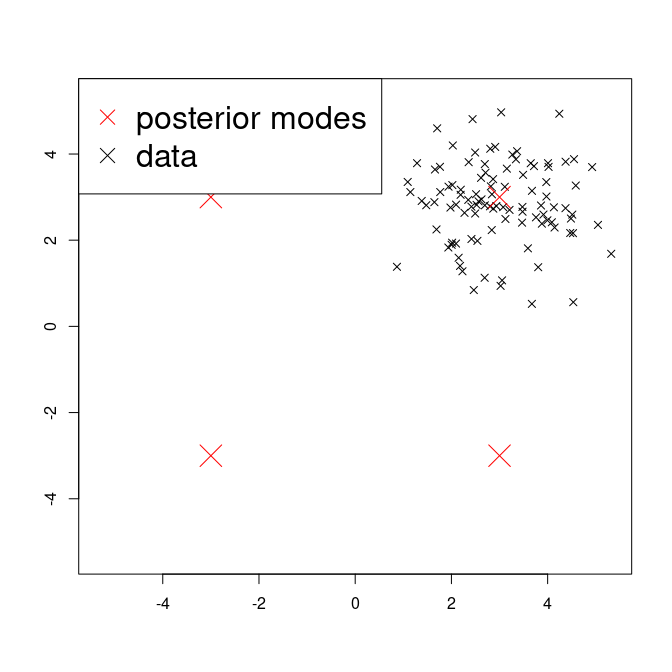Now for some standard MCMC using nimble’s default choice of samplers.  simulate(cModel, "centroids") mcmcR <- buildMCMC(configureMCMC(cModel, nodes="centroids", monitors="centroids"), print=TRUE) #> ===== Monitors ===== #> thin = 1: centroids #> ===== Samplers ===== #> RW sampler (2) #> - centroids[] (2 elements) mcmcC <- compileNimble(mcmcR) #> Compiling #> [Note] This may take a minute. #> [Note] Use 'showCompilerOutput = TRUE' to see C++ compilation details. mcmcC$run(niter=15000)
#> |-------------|-------------|-------------|-------------|
#> |-------------------------------------------------------|
#> NULL

samples <- tail(as.matrix(mcmcC$mvSamples), 10000) summary(samples) #> centroids centroids #> Min. :-3.320 Min. :-3.245 #> 1st Qu.:-3.056 1st Qu.:-2.919 #> Median :-2.983 Median :-2.851 #> Mean :-2.985 Mean :-2.851 #> 3rd Qu.:-2.916 3rd Qu.:-2.783 #> Max. :-2.529 Max. :-2.530 plot(samples, xlab="", ylab="", typ="l", xlim=c(-1,1)*max(cModel$y), ylim=c(-1,1)*max(cModel$y)) points(x=cModel$centroids, y=cModel$centroids, col="red", pch=4, cex=3) points(x=-cModel$centroids, y=cModel$centroids, col="red", pch=4, cex=3) points(x=cModel$centroids, y=-cModel$centroids, col="red", pch=4, cex=3) points(x=-cModel$centroids, y=-cModel$centroids, col="red", pch=4, cex=3) legend("topleft", legend=c("posterior modes","jumps"), col=c("red","black"), pch=c("X","_"), bg="white")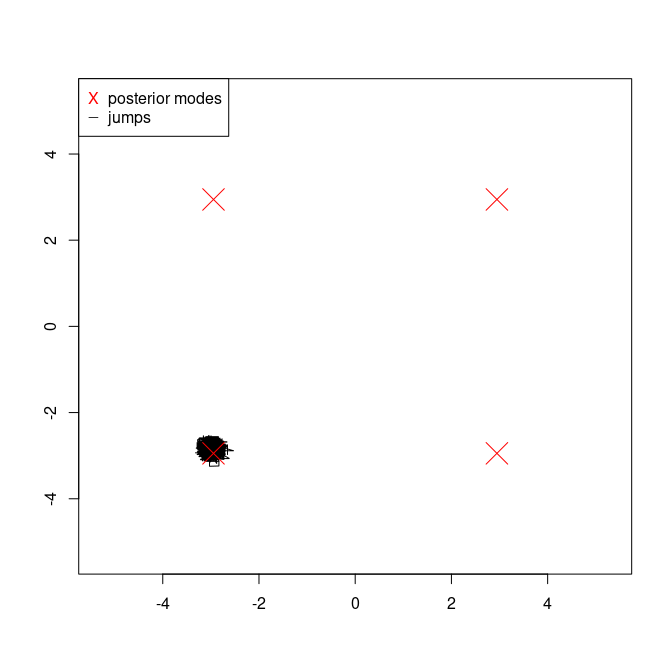library(coda) # Loads coda plot(as.mcmc(samples))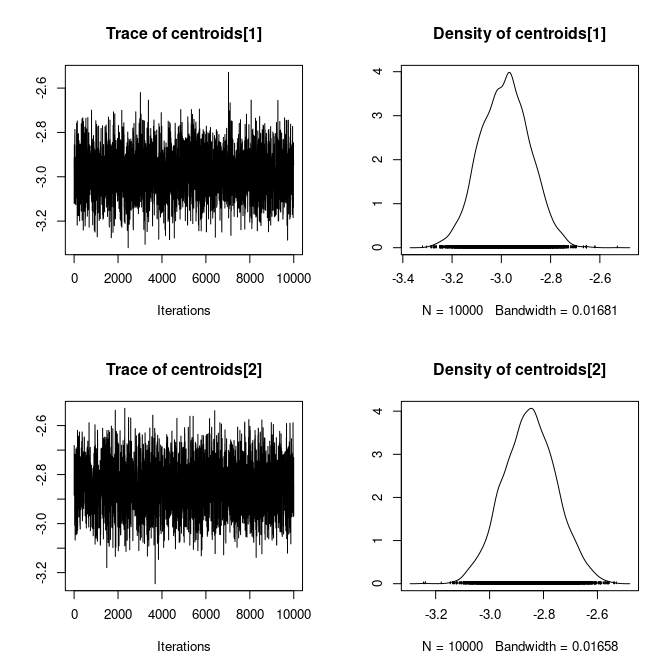As we can see, the default MCMC scheme makes few, if any, jumps between the four potential modes. So let’s try with adaptive parallel tempering (APT).  conf <- configureMCMC(cModel, nodes="centroids", monitors="centroids", enableWAIC = TRUE) #> ===== Monitors ===== #> thin = 1: centroids #> ===== Samplers ===== #> RW sampler (2) #> - centroids[] (2 elements) conf$removeSamplers()
conf$addSampler("centroids", type="sampler_RW_tempered", control=list(temperPriors=TRUE)) conf$addSampler("centroids", type="sampler_RW_tempered", control=list(temperPriors=TRUE))
conf
#> ===== Monitors =====
#> thin = 1: centroids
#> ===== Samplers =====
#> RW_tempered sampler (2)
#>   - centroids[]  (2 elements)

aptR <- buildAPT(conf, Temps=1:5, ULT= 1000, print=TRUE)
#> Initial temperatures: 1 2 3 4 5
#> Monitored nodes are valid for WAIC

aptC <- compileNimble(aptR)
#> Compiling
#>   [Note] This may take a minute.
#>   [Note] Use 'showCompilerOutput = TRUE' to see C++ compilation details.

aptC$run(niter=15000) #> |-------------|-------------|-------------|-------------| #> |-------------------------------------------------------| #> NULL samples <- tail(as.matrix(aptC$mvSamples), 10000)
summary(samples)
#>   centroids      centroids
#>  Min.   :-3.3436   Min.   :-3.22573
#>  1st Qu.:-2.9542   1st Qu.:-2.85378
#>  Median : 2.8919   Median : 2.66572
#>  Mean   : 0.5712   Mean   : 0.09863
#>  3rd Qu.: 3.0110   3rd Qu.: 2.85551
#>  Max.   : 3.3882   Max.   : 3.19312

plot(samples, xlab="", ylab="", typ="l", xlim=c(-1,1)*max(cModel$y), ylim=c(-1,1)*max(cModel$y))
points(samples, col="red", pch=19, cex=0.1)
legend("topleft", legend=c("jumps", "samples"), col=c("black","red"), pch=c("_","X"), bg="white")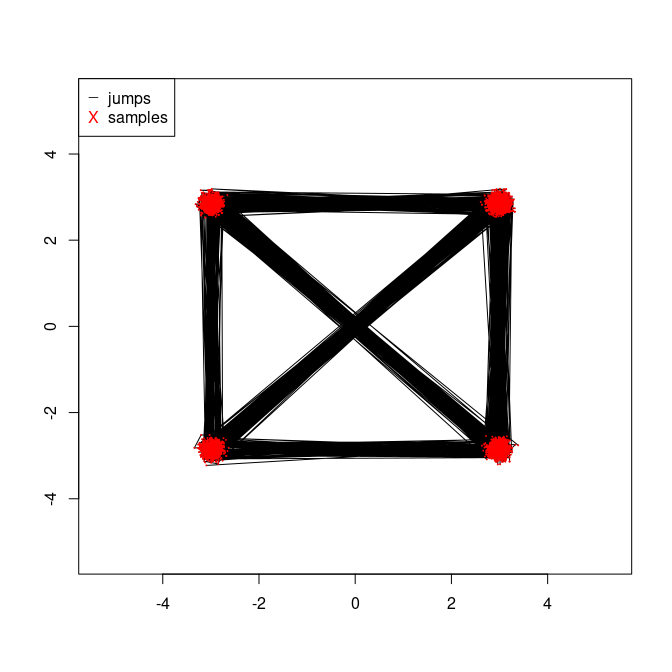plot(as.mcmc(samples))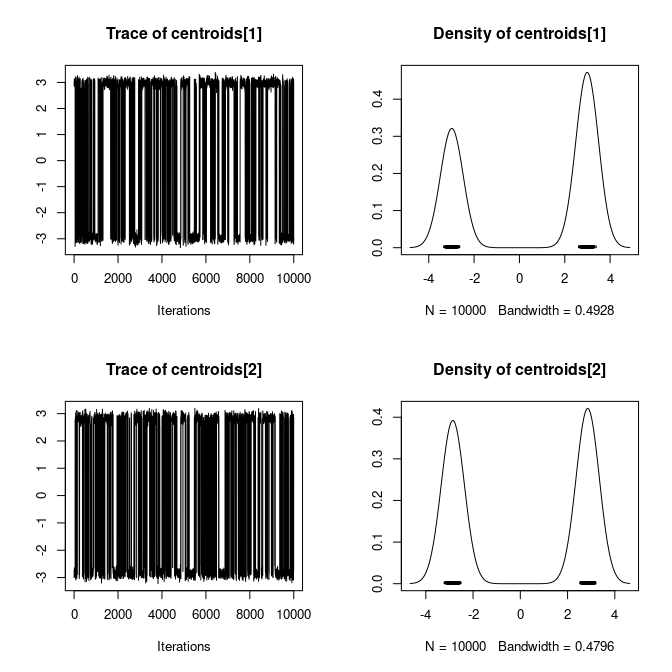plot(as.mcmc(aptC$logProbs))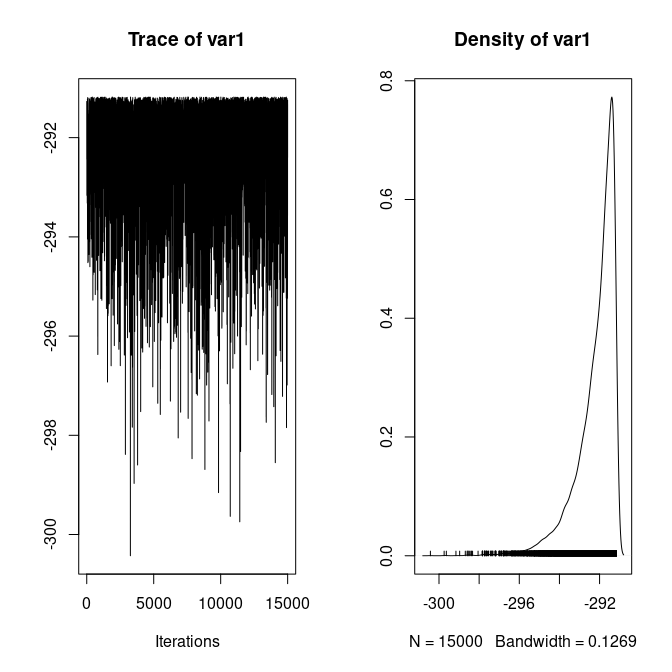aptC$calculateWAIC()
#>  554.8819

We can see that jumps between nodes (black lines) are frequent and that each node has been sampled (red points). The precision with which the weight of each posterior mode is estimated can be increased by increasing 1. the number of rungs in the temperature ladder, and moreover 2. the number of iterations (niter).

Finally, note that WAIC can be computed in exactly the same way as in nimble. See the section ‘calculating WAIC’ in the nimble manual for further details.